# Excel查找匹配函数的16种方法

2019/04/10 10:10

1、普通查找

=VLOOKUP(H2,B:F,5,0)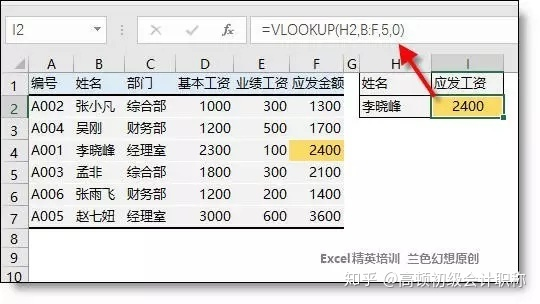2、反向查找

=INDEX(A:A,MATCH(H2,B:B,0))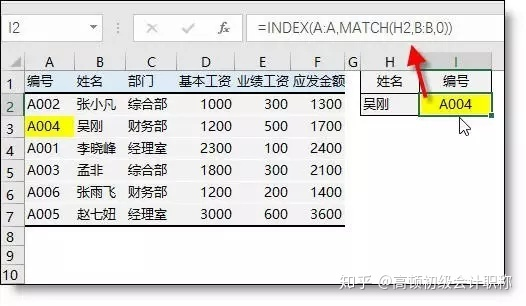3、交叉查找

=VLOOKUP(H2,A:F,MATCH(I2,1:1,0),0)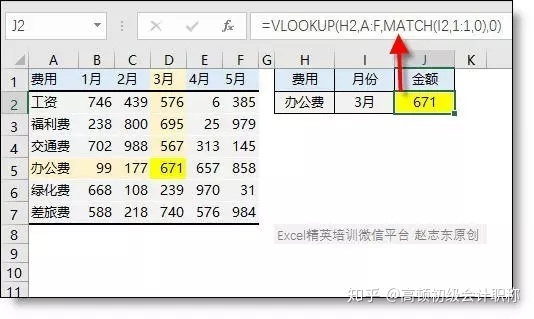4、多条件查找

=LOOKUP(1,0/((A2:A7=E2)*(B2:B7=F2)),C2:C7)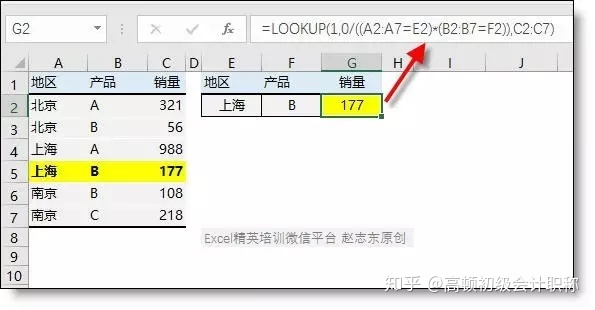5、区间查找

=LOOKUP(A2,$D$2:$E$5)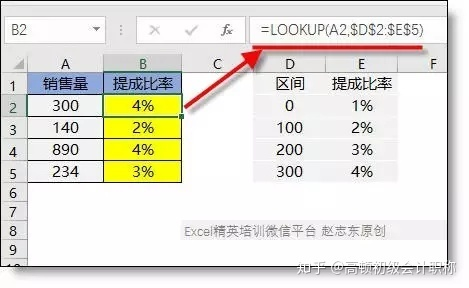6、双区间查找

=INDEX(B3:F7,MATCH(D11,A3:A7),MATCH(E11,B2:F2))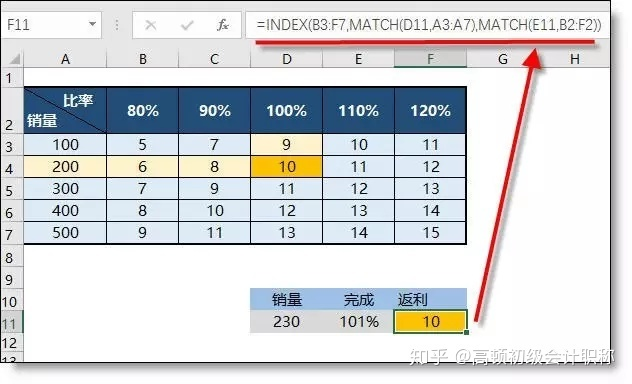7、线型插值

=TREND(OFFSET(B1,MATCH(D3,A2:A6,1),,2,1),OFFSET(A1,MATCH(D3,A2:A6,1),,2,1),D3)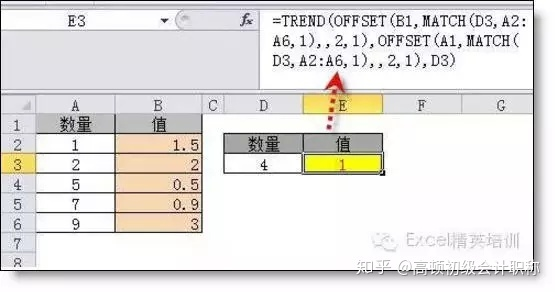8、查找最后一个符合条件记录

=LOOKUP(1,0/(B2:B9=A13),C2:C9)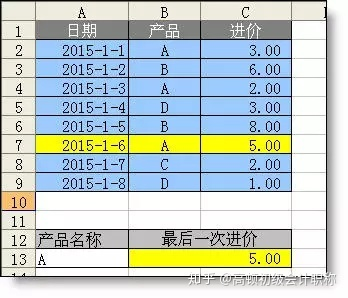9、模糊查找

=VLOOKUP("*"&A7&"*",A1:B4,2,0)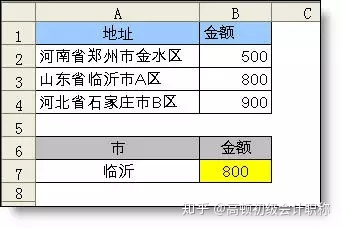10、匹配查找

=lookup(9^9.find(A$3:A$6,A10),B$3:B$6)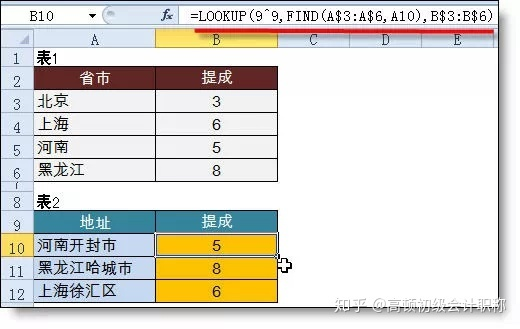11、最后一个非空值查找

=LOOKUP(1,0/(B2:B13<>""),$A2:$A13)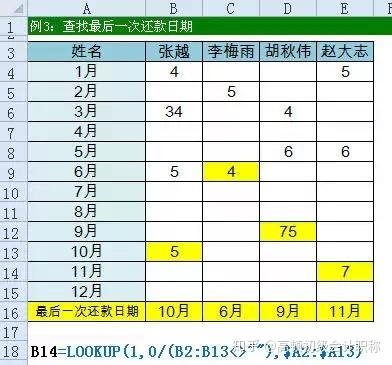12、多工作表查找

【例10】从各部门中查找员工的基本工资，在哪一个表中不一定。

=IFERROR(VLOOKUP(A2,服务!A:G,7,0),IFERROR(VLOOKUP(A2,人事!A:G,7,0),IFERROR(VLOOKUP(A2,综合!A:G,7,0),IFERROR(VLOOKUP(A2,财务!A:G,7,0),IFERROR(VLOOKUP(A2,销售!A:G,7,0),"无此人信息")))))

=VLOOKUP(A2,INDIRECT(LOOKUP(1,0/COUNTIF(INDIRECT({"销售";"服务";"人事";"综合";"财务"}&"!a:a"),A2),{"销售";"服务";"人事";"综合";"财务"})&"!a:g"),7,0)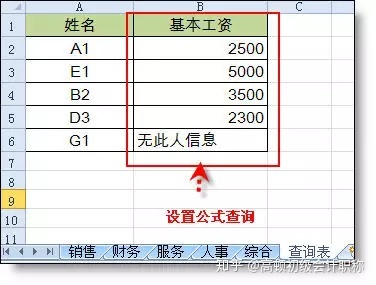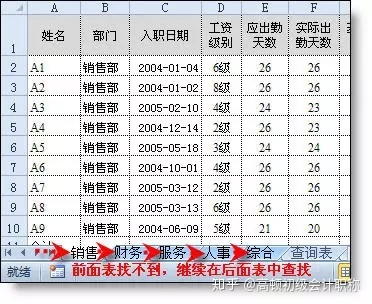13、一对多查找

【例】根据产品查找相对应的所有供应商

A2 =B2&COUNTIF(B$1:B2,B2) B11=IFERROR(VLOOKUP($A11&COLUMN(A1),$A:$C,3,0),"")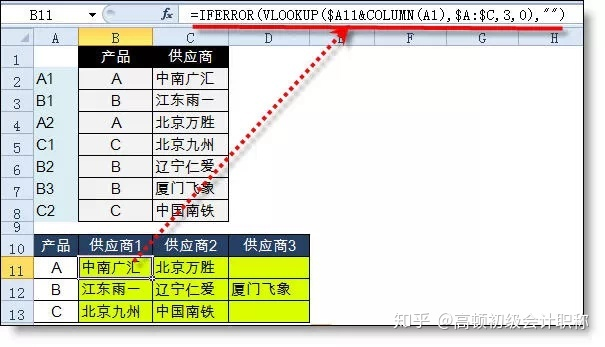14、查找销量最大的城市

{=INDEX(A:A,MAX((MAX(B3:B7)=B3:B7)*ROW(B3:B7)))}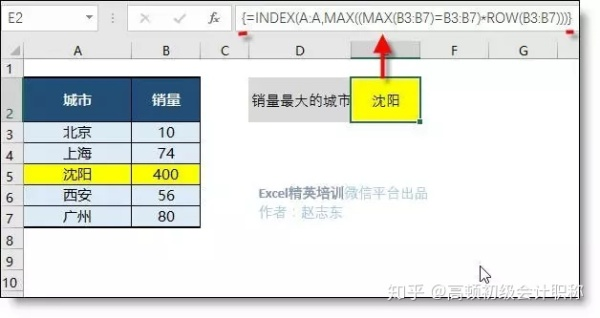15、最接近值查找

（数组公式按ctrl+shift+enter三键输入）

{=LOOKUP(1,0/(MIN(ABS(B3:B7-D4))=ABS(B3:B7-D4))*ROW(B3:B7),A3:A7)}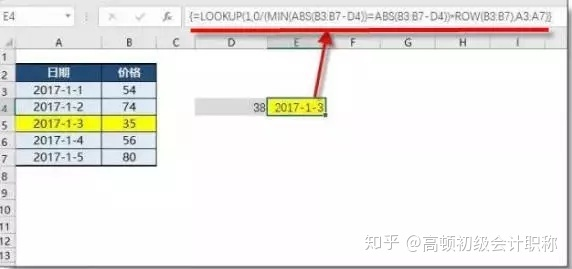15、跨多文件查找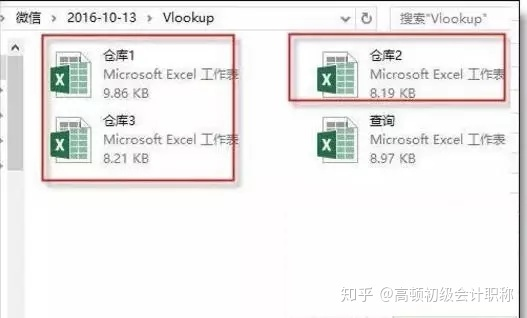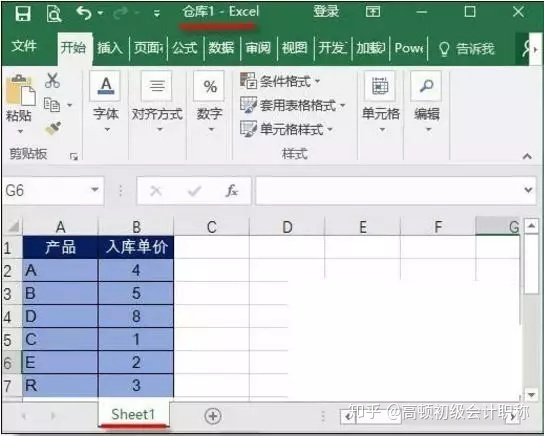=VLOOKUP(A2,INDIRECT(LOOKUP(1,0/COUNTIF(INDIRECT("["&{"仓库1";"仓库2";"仓库3"}&".xlsx]sheet1!a:a"),A2),"["&{"仓库1";"仓库2";"仓库3"}&".xlsx]sheet1")&"!a:b"),2,0)。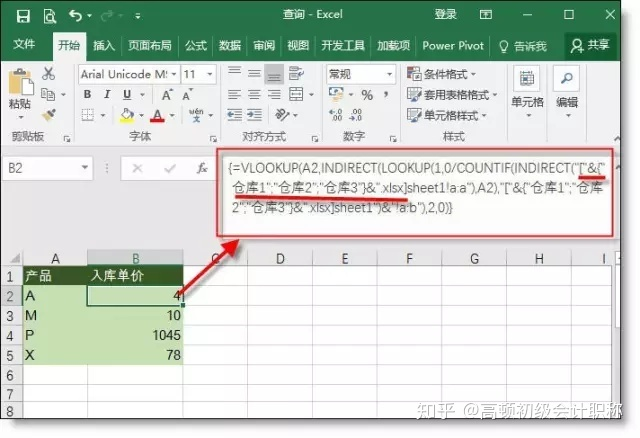vlookup函数的多文件查找，同样可以用iferror+vlookup的模式，公式虽然长，但容易理解且公式不容易出错。另外，如果excel文件有几十个或更多，就需要使用宏表函数用Files获取所有excel文件名称，然后应用到公式中，具体的公式小编就不再写了。

0
0 收藏

### 作者的其它热门文章0 评论
0 收藏
0